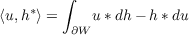#### Vol. 93, No. 1, 1981

 Recent Issues Vol. 325: 1 Vol. 324: 1  2 Vol. 323: 1  2 Vol. 322: 1  2 Vol. 321: 1  2 Vol. 320: 1  2 Vol. 319: 1  2 Vol. 318: 1  2Online Archive Volume: Issue:The Journal Subscriptions Editorial Board Officers Contacts Submission Guidelines Submission Form Policies for Authors ISSN: 1945-5844 (e-only) ISSN: 0030-8730 (print) Special Issues Author Index To Appear Other MSP Journals
Harmonic functionals on open Riemann surfaces

### Mitsuru Nakai and Leo Sario

Vol. 93 (1981), No. 1, 147–161
##### Abstract

We denote by H(R) the linear space of harmonic functions on an open Riemann surface R with the topology of uniform convergence on every compact subset. A continuous linear functional on H(R) is referred to as a harmonic functional on R; the totality of such functionals is the dual space H(R) of the locally convex space H(R). A point evaluation u u(z), with z a fixed point of R; and a period u γ du, with γ a fixed cycle on R, are the most common examples of harmonic functionals frequently occurring in the theory of functions. We denote by u,hthe value of a harmonic functional h on R at u in H(R). The main purpose of the present study is to establish the following representation of harmonic functionals:

Representation Theorem. Every harmonic functional h on an open Riemann surface R can be represented by means of a function h harmonic at the point at infinity of R as(1)

for every u in H(R), where W is any relatively compact subregion of R such that the relative boundary ∂W is smooth and h is harmonic on R W. If h1 and h2 are functions representing h in the above sense, then h1 h2 can be continued harmonically to all of R.

Primary: 30F15
Secondary: 31C05
##### Milestones